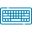# Triple Integral CalculatorLimit for x

Limit for y

Limit for Z

## Table of Content

Feedback

The online triple integral calculator finds the triple integrand values of the given function. The cylindrical integral calculator finds the volume of three-dimensional objects with steps.

## What is Triple Integral?

In Calculus:

“The analog of double integrals for three dimensions is known as a triple integral”.

It is the same concept as the single and the double integration. It is used to determine the volume like double integrals.

## Triple Integral Formula:

The integration of functions for the three variables can be calculated by the below formula that is considered by the triple integrals calculator. These functions of variables can be represented over the three-dimensional space by w=f(x,y,z).

The following formula is used at the time of triple integration calculation.

$$\int_{z_1}^{z_2} \left( \int_{y_1}^{y_2} \left( \int_{x_1}^{x_2} f(x,y,z)dx \right) dy \right) dz$$

In order to determine the function with the triple iterated integral calculator or to find it manually you can take into service the following points:

• Choose the function that has three different variables like x, y, z
• To eliminate certain variables perform one variable integration and consider others as constant
• Replace the values in the upper and lower limits of the expression

## How To Evaluate The Triple Integral?

In order to solve the integrations of the variable functions by using the triple integral calculator the following example is the best approach that clarifies their concept.

### Example:

$$\int\limits_{0}^{3}\int\limits_{3}^{2}\int\limits_{0}^{1} \left(x^{3} + 5 x y z^{2} + x y z\right)\, dx\, dy\, dz$$

#### Solution:

Indefinite Integral

$$=\frac{x^{2} y z \left(6 x^{2} + 10 y z^{2} + 3 y z\right)}{24}+ \mathrm{constant}$$

Definite Integral

$$= - \frac{501}{8}$$

Integral Steps:

• First, we take the inner integral:

$$\int \left(x^{3} + 5 x y z^{2} + x y z\right)\, dx$$

$$\frac{x^{4}}{4} + x^{2} \left(\frac{5 y z^{2}}{2} + \frac{y z}{2}\right)$$

• Now simplify:

$$\frac{x^{2} \left(x^{2} + 2 y z \left(5 z + 1\right)\right)}{4}$$

• Add the constant of integration:

$$\frac{x^{2} \left(x^{2} + 2 y z \left(5 z + 1\right)\right)}{4}+ \mathrm{constant}$$

$$\frac{x^{2} \left(x^{2} + 2 y z \left(5 z + 1\right)\right)}{4}+ \mathrm{constant}$$

• Then we take the second integral:

$$\int \frac{x^{2} \left(x^{2} + 2 y z \left(5 z + 1\right)\right)}{4}\, dy$$

The integral of a constant times a function is the constant times the integral of the function:

$$\int \frac{x^{2} \left(x^{2} + 2 y z \left(5 z + 1\right)\right)}{4}\, dy = \frac{x^{2} \int \left(x^{2} + 2 y z \left(5 z + 1\right)\right)\, dy}{4}$$

• Integrate term-by-term:

The integral of a constant is the constant times the variable of integration:

$$\int x^{2}\, dy = x^{2} y$$

The integral of a constant times a function is the constant times the integral of the function:

$$\int 2 y z \left(5 z + 1\right)\, dy = 2 z \left(5 z + 1\right) \int y\, dy$$

• The integral of yn is:

$$\frac{y^{n + 1}}{n + 1}$$ when n≠−1:

$$\int y\, dy = \frac{y^{2}}{2}$$

• So, the result is:

$$y^{2} z \left(5 z + 1\right)$$

$$x^{2} y + y^{2} z \left(5 z + 1\right)$$

So, the result is: $$\frac{x^{2} \left(x^{2} y + y^{2} z \left(5 z + 1\right)\right)}{4}$$

Now simplify: $$\frac{x^{2} y \left(x^{2} + y z \left(5 z + 1\right)\right)}{4}$$

• Add the constant of integration:

$$\frac{x^{2} y \left(x^{2} + y z \left(5 z + 1\right)\right)}{4}+ \mathrm{constant}$$

The answer is: $$\frac{x^{2} y \left(x^{2} + y z \left(5 z + 1\right)\right)}{4}+ \mathrm{constant}$$

• At the end, we take the third integral:

$$\int \frac{x^{2} y \left(x^{2} + y z \left(5 z + 1\right)\right)}{4}\, dz$$

$$\frac{x^{4} y z}{4} + \frac{5 x^{2} y^{2} z^{3}}{12} + \frac{x^{2} y^{2} z^{2}}{8}$$

• Now simplify:

$$\frac{x^{2} y z \left(6 x^{2} + 10 y z^{2} + 3 y z\right)}{24}$$

• Add the constant of integration:

$$\frac{x^{2} y z \left(6 x^{2} + 10 y z^{2} + 3 y z\right)}{24}+ \mathrm{constant}$$

The answer is: \frac{x^{2} y z \left(6 x^{2} + 10 y z^{2} + 3 y z\right)}{24}+ \mathrm{constant}

## Working of Triple Integral Calculator:

It is extremely helpful to use the triple integral solver if you need fast results of analog for double integrals for three dimensions.

Input:

• Select the option from definite and indefinite
• Put the functions with variables like x, y, and z
• Chose the substitute upper and lower limit
• Put the values of the limit for x, y, and z
• Tap on “Calculate”

Output:

An online triple integral calculator with steps gives you the final results as follows:

• Definite integrals for the given inputs
• Indefinite integrals
• Complete calculations with steps

## Integration in Cylindrical Coordinates:

To perform the triple integrals in the cylindrical coordinates to cartesian coordinates some equations are used that are listed below in the table.

 Circular Cylinder Circular cone Sphere Paraboloid Cylindrical R = c Z = cr $$R^2 + z^2 = c^2$$ $$Z = cr^2$$ Rectangular $$X^2 + y^2 = c^2$$ $$Z^2 = c^2 (x^2 + y^2)$$ $$X^2 + y^2 + z^2 = c^2$$ $$Z = c(x^2 + y^2)$$

## Triple Integrals over a General Bounded Region:

Over a more general bounded region to compute the triple integral we take into service all three types

E={(x,y,z)|(x,y)∈D,u1(x,y)≤z≤u2(x,y)}.

For two functions z=u1(x,y) and u2(x,y), such that u1(x,y)≤u2(x,y) for all (x,y) in D as shown in the following figure.## References:

From the source Wikipedia: Triple integral

From the source Libretext: Triple Integrals, Triple Integrals over a General Bounded Region, Triple Integrals in Cylindrical Coordinates### Shaun Murphy

I'm graduated in biomedical and electrical engineering. Specialization in sports and medical topics but will gladly tackle everything you throw at him. He is a sailor, hiker, and motorcyclist in his free time.